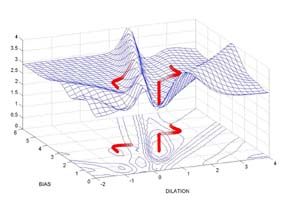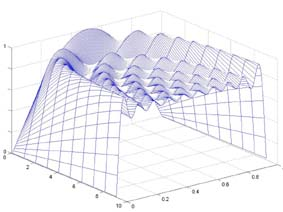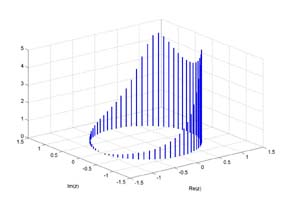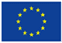Gradient methodsFourier seriesFunctional transforms

SOCRATES EU Programmme ATHENSDigital Signal and Image Processing with Applications

BACHALOR'S STUDY PROGRAMME - subjects

1. Mathematical Methods in Engineering
2. Signal Processing
3. Multimedia Processing

MASTER'S STUDY PROGRAMME - subjects

4. Digital Signal and Image Processing
5. Neural Networks

POSTGRADUATE STUDY PROGRAMME - selected research topics of dissertations

1. Linear and Non-Linear Models in Signal Prediction
2. Biomedical Signal and Image Processing
3. Signal and Image Analysis and Processing in Environmental Systems
4. Biomedical Image Processing

MATHEMATICAL METHODS IN ENGINEERING
1. Algorithmic tools of computing methods, MATLAB programming environment
2. Numerical and visualisation methods, object graphics, programming tools
3. Symbolic methods in engineering
4. Modelling and simulation in the Simulink environment
5. Numerical and symbolical methods of linear algebra, sparse matrices
6. Data processing, linear approximation, the least square method, modelling tools
7. Nonlinear approximation, gradient method, numerical optimisation
8. Algorithmic tools for solution of nonlinear equations, iterative algorithms, symbolic methods
9. Methods of numerical and symbolic interpolation, differentiation and integration
10. Numerical and symbolical methods of solution of ordinary differential equations, modelling
11. Systems of ordinary differential equations, boundary value problems, modelling in Simulink
12. Modeling of dynamical systems, selected numerical and visualisation blocks, Simulink
13. Remote data processing, MATLAB WWW server
14. Computer project

TOP

SIGNAL PROCESSING
1. Sampling of continuous signals, processing of physical measurements
2. Technical and programming tools of analog/digital conversion, Simulink environment
3. Remote communication with a system, application of Matlab Web server
4. Wireless communication, data transmition, resolution
5. Description of one-dimensional and two-dimensional signals in time domain, visualization
6. Signal representation in frequency domain, discrete Fourier transform
7. Spectral analysis, short-time Fourier transform
8. System modelling and data analysis, application of Simulink
9. System description in time domain, difference equations, impulse and step response
10. Discrete transfer function, stability
11. Frequence characteristics, basic properties
12. Fundamental methods of digital filtering in time domain, moving average
13. Principle of FIR and IIR filters, basic properties
14. System modelling and time series prediction

TOP

DIGITAL SIGNAL AND IMAGE PROCESSING
1. Algorithmic tools of digital signal processing, fundamentals of MATLAB environment
2. Numerical, symbolic and visualization tools of MATLAB, data files processing
3. Time-domain signal representation, difference equations, selected statistical methods
4. Frequency-domain signal analysis, sampling, discrete Fourier transform, decomposition
5. Spectrum estimation, aliasing, short-time Fourier transform, window functions
6. Z-transform and system description, discrete transfer function, frequency transfer function
7. Digital filters, basic methods in the time domain, convolution, FIR filters, filter banks
8. IIR filters, basic properties, signal decimation
9. Filtering methods in frequency domain, signal reconstruction, window functions
10. Linear methods of time series modelling and prediction, SVD a QR algorthm, model selection
11. Nonlinear methods of signal processing, median filters, principles of neural networks
12. Basic methods of image analysis, 2D Fourier transform, image processing
13. Signal processing in engineering, signal prediction, Simulink environment, basic blocks
14. Biomedical signal and image processing

TOP

NEURAL NETWORKS
1. Fundamentals of MATLAB environment, basic operations, data files processing
2. Visualization tools in MATLAB, symbolic mathematics, principles of SIMULINK
3. Basic mathematical models of neurons, transfer functions, threshold, error surface evaluation
4. Single-layer networks, perceptron learning rule, application to classification problems
5. Adaptive linear networks, Widrow-Hoff learning rule, training and coefficients optimization
6. Neural networks in adaptive noise cancellation
7. Multi-layer networks, error function, optimization of parameters, gradient descent method
8. Basic optimization methods (backpropagation, Levenberg-Marquardt algorithm)
9. Neural networks in signal prediction and system modeling, learning and generalization
10. Radial basis networks, transfer functions definition, network structure optimization
11. Associative learning rules, self-organizing networks and maps, Hebian learning, Kohonen rule
12. Neural networks in segmentation, feature extraction and classification, competitive learning
13. Simulation of artificial neural networks in the SIMULINK environment
14. Neural networks in system identification and control, supervised and unsupervised learning

TOP

TOPICS OF POSTGRADUATE PROJECTS

LINEAR AND NON-LINEAR METHODS IN SIGNAL PREDICTION
The project goal is in the analysis of autoregressive models for prediction of time series, estimation of reliability limits followed by the study of principal components analysis and orthogonal decomposition to simplify resulting mathematical model. Results will be compared with that achieved by artificial neural networks. Application will be devoted to the gas consumption prediction.

TOP

BIOMEDICAL SIGNAL AND IMAGE PROCESSING
Project will be devoted to methods of signal and image analysis and to algorithms of their linear and nonlinear processing for rejection of their undesirable additive components, enhancement, detection of separate objects and their identification with applications to biomedical signals. Mathematical methods will include discrete transforms, methods of signal decomposition and reconstruction by wavelet transform, gradient method and application of interpolation methods.

TOP

SIGNAL AND IMAGE ANALYSIS AND PROCESSING OF ENVIRONMENTAL SIGNALS
Project will be devoted to methods of signal and image analysis, correlation analysis and rejection of additive noise signal components. The goal is in analysis and processing of environmental signals including satellite images. Mathematical tools include discrete transforms and selected statistical methods in the MATLAB environment.

TOP

BIOMEDICAL IMAGE PROCESSING
The project goal is in application of linear and nonlinear methods of biomedical image processing including methods of feature extraction, segmentation and classification to detect specific image components for the following three-dimensional modelling.

TOP